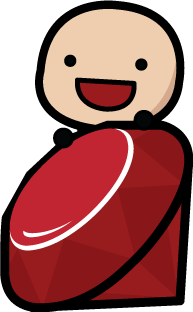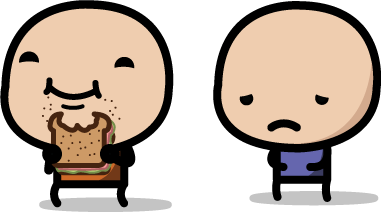# RubyKin## If and Else

“If you clean your room then you can play, or else you won’t.” - Mom

If and else statements help Ruby understand what you want her to do and when you want her to do it.

So far, we’ve learned how Ruby can perform basic math on numbers, store words and sentences as strings, and place these types of information into memory stored in variables. Now that we know how to store information and interact with it, we need a way of telling the computer what to do with that information.

One way we do that is with conditionals. This is why your parents say they love you unconditionally, because there is no if or else, there is only 100% love, no matter what. Ruby doesn’t love anything unconditionally, Ruby needs to be convinced by conditions.

A conditional is something that depends on other factors. If this something happens, do that, otherwise, do something else. For example, if you are hungry, eat a sandwich, else, don’t eat a sandwich!Art by Vixuong Hong

Here’s how an If Else conditional might look in Ruby.

``````# set an x variable to the value 5
x = 5

# start the if / else conditional
if x <= 10
puts x
else
puts "Number is greater than 10"
end
``````

In a conditional, the computer checks to see that the code after the if is true. We call this code a block, which just means a small piece of code that has some function. In this case, our block is `X <= 10`.

So, if our block is true (if X is less than or equal to 10), the computer will perform the action in the next line below the if statement. Since X is equal to 5, it is less than 10, and the value 5 is put on the screen. If X were 11, the conditional (X <= 10) would realize this was a false statement, and the string “Number is greater than 10” would be outputted (shown) on the screen.

When the computer moves through an if-else conditional, it will follow the instructions under the first if statement that evaluates to true. It then ignores all other conditions in the if-else conditional.

What is a boolean?

We’ve just learned how a conditional checks to see if something is `true` or `false`. A true or false value is called a boolean in programming. We could write another conditional in a different way using booleans.

``````if false
puts "false"
elsif true
puts "true"
else
puts "This won’t print"
end
``````

Let’s walk through each step to get a better understanding. `Nil` and `False` are the only objects in Ruby that are considered falsy. Something is falsy when it has a false value. `Nil` is Ruby’s way of representing nothing–nada, zip, zero! No value stored here whatsoever!

In the example above, the conditional checks to see if false is true. Since the `false` object will never be true, the computer moves on to the next line to see if `true` is true. It is, so Ruby puts the string true to the screen!

The final `else` will never print, because true will always evaluate to true and so the program exits before getting to the else line. Remember, Ruby is only looking for the first true if statement, ignoring everything else.

Here are the basic elements used to evaluate conditionals:

``````<   # less than
>   # greater than
<=  # less than or equal to
>=  # greater than or equal to
==  # equal to
!=  # not equal to
``````

When checking if an object is less than or greater than, we use the same symbols found in math. When checking if some object is equal to another object, we use two equal signs. In Ruby, like many programming languages, one equal sign is used to assign or give a value to a variable. If we want to check that an object is not equal we use an exclamation mark before the equal sign. In Ruby, we could also simply use the word `not`.

What’s an object?

You may have noticed that we keep talking about things as objects. To understand a code object think about an object in real life. A ball, a book, or even your dog–these are all different types of objects or physical things. In programming, we try to represent real life objects with code objects.

For example, if you walk the dogs in your neighborhood, you may know their names in your head. Rex, Sparky, and Spot. But how would a computer know their names? Well, we could represent each dog as a string object in Ruby, like so:

``````["Rex", "Sparky", "Spot"]
``````

In fact, this line of code is actually four objects! Can you see why? We have Rex, Sparky and Spot, that makes three objects. But we also have the list of dogs, which is another object! This is the neat thing about Ruby, everything is an object. Let me say that again.

__*Everything is an object*__

That means that every thing you have learned so far was a type of object. Numbers, strings, variables, if statements, booleans and even code blocks (such as X < 10)…these are all objects!

Next chapter we’ll learn about Loops. (Yep, loops are objects too). Don’t worry, these aren’t the roller coaster type of loops.

Practice

What would the computer say? Try to do these in your head before going to IRB. Remember, the answers will be a boolean value, either true or false.

``````1) Is 3 > 5 ?

2) 3 < 5

3) 5 == 5

4) 10 >= 10

5) 10 <= 12

6) 10 != 10

7) 10.object_id == 10.object_id

What about strings?
8) "dog" == "cat"

9) "cat" == "cat"

10) "cat.object_id" == "cat.object_id"
``````